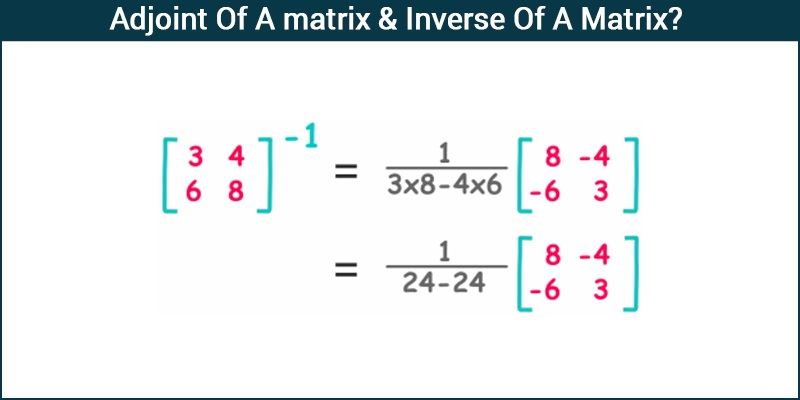# Adjoint Of A matrix & Inverse Of A Matrix?

Adjoint of a Matrix:

Let  A = $[a_{ij}]_{n×n}$ denote a square matrix. The adjoint of  A is the transpose of matrix obtained by replacing each element of A by its cofactor in |A|. It is denoted as adj A = $[b_{ij}]_{n×n} b_{ij}$ = $A_{ji}$  where  $A_{ji}$ represents the cofactor of the $(j,i)^{th}$  element of the matrix A. In simple termsthe transpose of the cofactor matrix of  is known as the adjoint of A or adj A.

Let A = $\left[\begin{matrix} a_{11} & a_{12} & a_{13}\cr a_{21} & a_{22} & a_{23} \cr a_{31} & a_{32} & a_{33}\cr \end{matrix}\right]$

Then adj A = $\left[\begin{matrix} A_{11} & A_{12} & A_{13}\cr A_{21} & A_{22} & A_{23} \cr A_{31} & A_{32} & A_{33}\cr \end{matrix}\right]^T$

Where $\left[ \begin{matrix} A_{11} & A_{12} & A_{13}\cr A_{21} & A_{22} & A_{23} \cr A_{31} & A_{32} & A_{33}\cr \end{matrix} \right]^T$
represent the cofactor matrix.

$~~~~~~~~~~~~~~~$ adj A = $\left[\begin{matrix} A_{11} & A_{12} & A_{13}\cr A_{21} & A_{22} & A_{23} \cr A_{31} & A_{32} & A_{33}\cr \end{matrix}\right]$1. For a square matrix A of order n the following property holds true

A ( adj A ) = (adj A) A = |A| In , where In  is an identity matrix of order n .

2. adj kA = $k^{n-1}$ ( adj A )

3. adj (AB) = ( adj B) (adj A )

For a better understanding let us take an example.

Example:Find adj A for  A = $\left[\begin{matrix} 4 & -3 & 5\cr 1 & 0 & 3 \cr -1 & 5 & 2 \cr \end{matrix}\right]$

Solution: The cofactors of A  are

$C_{11}$$\left[\begin{matrix} 0 & 3 \cr 5 & 2 \cr \end{matrix} \right]^T$
= (0 – 15) = -15

Similarly we can calculate the other cofactors which come out to be

C12 = – 5

C13 = 5

C21 = 31

C22 = -13

C23 = -17

C31 = -9$C_{31}$ = – 9

C32 = -7

C33 = 3

Since the transpose of the cofactor matrix of A is adj A

⇒ adj A = $\left[\begin{matrix} -15 & -5 & 5\cr 31 & 13 & -17 \cr -9 & -7 & 3 \cr \end{matrix}\right]^T$

⇒ adj A = $\left[\begin{matrix} -15 & 31 & -9\cr -5 & 13 & -7 \cr 5 & -17 & 3 \cr \end{matrix}\right]$

Let us see what happens if the adjoint is multiplied by matrix .

A × adj A = $\left[\begin{matrix} 4 & -3 & 5\cr 1 & 0 & 3 \cr -1 & 5 & 2 \cr \end{matrix}\right]$

$\left[\begin{matrix} -15 & 31 & -9\cr -5 & 13 & -7 \cr 5 & -17 & 3 \cr \end{matrix}\right]$

We get A × adj A = $\left[\begin{matrix} -20 & 0 & 0\cr 0 & -20 & 0 \cr 0 & 0 & -20 \cr \end{matrix}\right]$

⇒ A × adj A = (-20) $\left[\begin{matrix} 1 & 0 & 0\cr 0 & 1 & 0 \cr 0 & 0 & 1 \cr \end{matrix}\right]$

Also det A = -20

So this justifies the property of the determinant which states that A(adj A) = (adj A)A = |A| In

Inverse of a matrix:

We have learnt that a square matrix A is called as singular if |A| = 0 and it is called as non-singular if |A|≠ 0.

Inverse of a square matrix A = $[a_{ij}]_{n×n}$ (which is denoted as $A^{-1}$) is the matrix  B such that

B = $[b_{ij}]_{n × n}$ such that AB = BA = In

In simpler terms the product of adj A with $\frac {1}{|A|}$ gives the inverse of a matrix A.

Inverse of a matrix if it exists is unique.

Since AB = In

Then using determinants

|AB| = |I|

$\Rightarrow |A||B|$ = 1d|I| = 1 )

1. d)

This indicates that |A| ≠ 0. Therefore A is non-singular.

Now as we know, A(adj A) = ( adj A)A = |A|In (By property of adjoint)

$\Rightarrow \frac {1}{|A|} (A(adj A))$= $\frac {1}{|A|}(adj A)A$ = I ————— (1)

Since AB = BA = I, from equation 1 we can say that B = $\frac {1}{|A|}$ adj A..

B represents the inverse of the matrix A and is given as B = $A^{-1}$ = $\frac {1}{|A|} adj A$ .

Let us solve the example given above to find out the inverse.

Example:Find $A^{-1}$ for A = $\left[\begin{matrix} 4 & 3 & 5\cr 1 & 0 & 3 \cr -1 & 5 & 2 \cr \end{matrix}\right]$

We have already figured out the adjoint of the given matrix which is

adj A = $\left[\begin{matrix} -15 & -31 & -9\cr 5 & 13 & 7 \cr 5 & 17 & 3 \cr \end{matrix}\right]$

Now for determining the inverse of the given matrix let us find its determinant

|A|= -20

As we know, $A^{-1} = \frac {1}{|A|} adj A$

We get, $A^{-1} = \frac {1}{20} \left[\begin{matrix} -15 & -31 & -9\cr 5 & 13 & 7 \cr 5 & 17 & 3 \cr \end{matrix}\right]$

This gives the required inverse of the given matrix A.

Download our app-BYJU’S the learning app to get acquainted to more concepts like Matrices, Determinants, and to study in an effective way.#### Practise This Question

If A is a square matrix of order 3 such that |A|=2 then     (adj A1)1 is